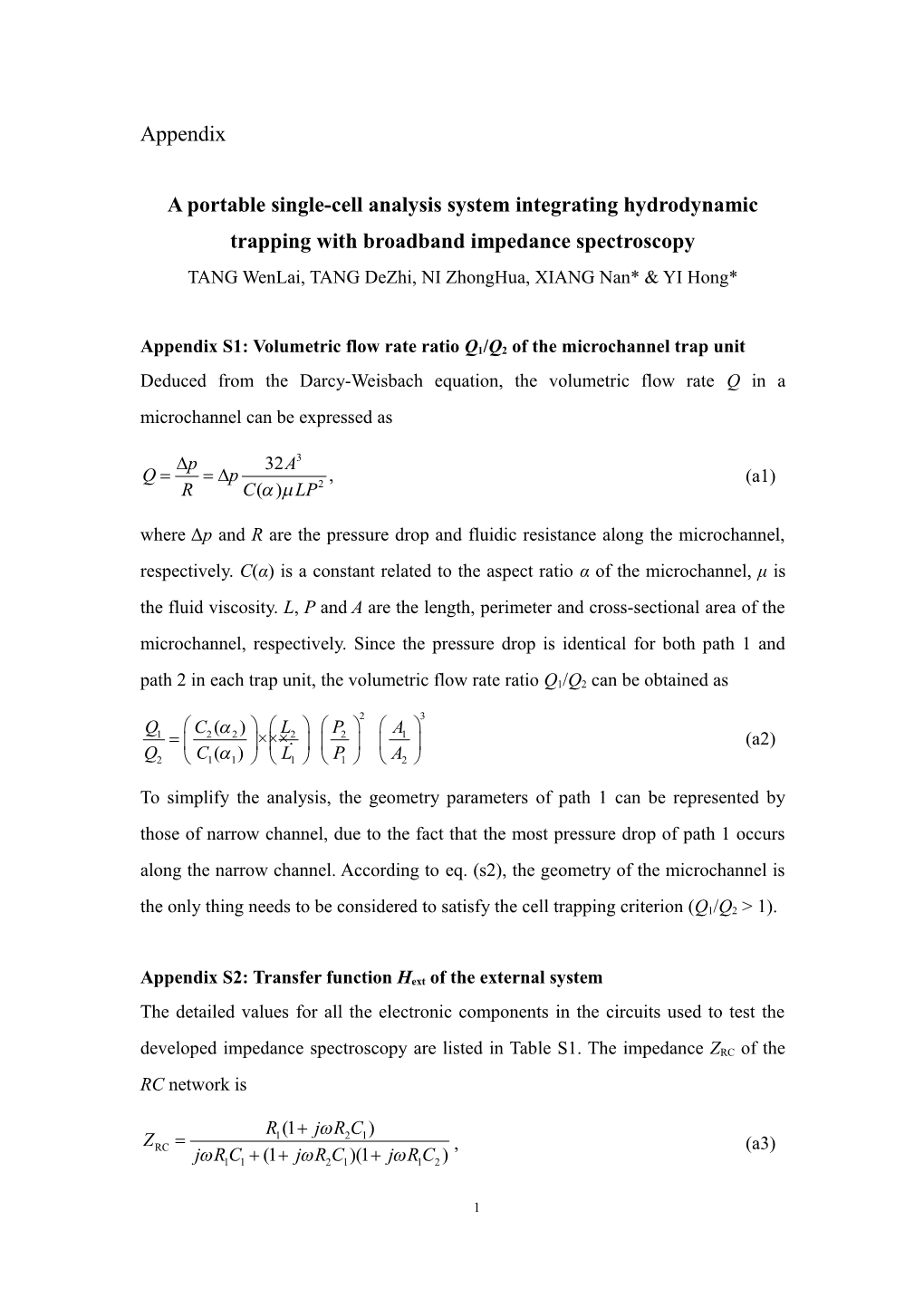# TANG Wenlai, TANG Dezhi, NI Zhonghua, XIANG Nan* & YI Hong*Appendix

A portable single-cell analysis system integrating hydrodynamic trapping with broadband impedance spectroscopy

TANG WenLai, TANG DeZhi, NI ZhongHua, XIANG Nan* & YI Hong*

Appendix S1: Volumetric flow rate ratio Q1/Q2 of the microchannel trap unit

Deduced from the Darcy-Weisbach equation, the volumetric flow rate Q in a microchannel can be expressed as

(a1)

where Δp and R are the pressure drop and fluidic resistance along the microchannel, respectively. C(α) is a constant related to the aspect ratio α of the microchannel, μ is the fluid viscosity. L, P and A are the length, perimeter and cross-sectional area of the microchannel, respectively. Since the pressure drop is identical for both path 1 and path 2 in each trap unit, the volumetric flow rate ratio Q1/Q2 can be obtained as

(a2)

To simplify the analysis, the geometryparameters of path 1 can be represented by those of narrow channel, due to the fact that the most pressure drop of path 1 occurs along the narrow channel. According to eq. (s2), the geometry of the microchannel is the only thing needs to be considered to satisfy the cell trapping criterion (Q1/Q2 > 1).

AppendixS2: Transfer function Hext of the external system

The detailed values for all the electroniccomponents in the circuits used to test thedeveloped impedance spectroscopy are listed in Table S1.The impedance ZRC of the RC network is

,(a3)

where j2 = −1 is the imaginary unit and ω is the angular frequency. The impedance Zsof the sensing circuit can be calculated as

(a4)

Replacingjωterms with theoperator s, the transfer function Hext(s)of the external system can be expressed as

(a5)

where a1= RDAQR1R2C1C2, a2 = RDAQ(R1C1+R2C1+R1C2), a3 = RDAQ, b1 = RDAQR1R2C1(C2+Cstray+CDAQ), b2 = RDAQR1C1+RDAQR2C1+RDAQR1C2+RDAQR1CDAQ+RDAQR1Cstray+R1R2C1, b3 = RDAQ+R1.

TableS1 Detailed values for all the electroniccomponents.

Component / R1 / R2 / C1 / C2 / RDAQ / CDAQ / Cstray
Value / 200 kΩ / 200 kΩ / 100 pF / 100 pF / 100 GΩ / 10 pF / 250 pF

AppendixS3: Time-dependent response Yext(t) of the external system

The bipolar MLS signal from the D/A converter can be expressed as

(a6)

where U0(t) is the unit step function, tirepresents when the polarity of the signal changes. Applying the Laplace transform to XMLS(t), the excitation MLS signal becomes

(a7)

Then the responseYext(s) of the external system can be expressed as

,(a8)

whereYext0(s)is the output response of the externalsystem under the unit step excitationU0(t), and can be given by

(a9)

By using the inverse Laplace transform to the Yext(s), the output response of the externalsystem acquired by DAQ card in the continuoustime domain can be obtained as

(a10)

with, where ki and si (i = 1, 2, 3, 4) are the residues and poles of Yext0(s).

Appendix S4:The complex impedance of the tested systemmeasured by sensing electrodes

The complex impedance Zmix of the cell mixture systemcan be expressed as

(a11)

witha4 = 2(Rleak + Rch), a5 = (RiRleakCmem + RiRchRmem + RleakRchCmem), b4 = 2 −ω2(RiRleakCmemCch + RiRchCmemCch + RleakRchCmemCch), b5 = 2RleakCch + 2RchCch + RiCmem+ RleakCmem). The complex impedance Zmed of the pure PBS solution can be expressed as

(a12)Figure S1 (Color online) Finite element simulations showing the flow velocity profiles in a trapping unit without cell and with a trapped cell. The laminar flow module in COMSOL Multiphysics is used. The flow is assumed to be uncompressible, inlet velocity is set to be 100 μm/s, outlet pressure is set to be 0 Pa, walls are set to the ‘No slip’ wall boundary condition. The fluid-flow simulation solver is based on the Navier-Stokes equations. An insulating sphere (15 μm diameter) is included to consider the influence caused by the trappedcell.Figure S2 (Color online) (a) Transfer functionHi of the internal circuit of DAQ card, which is measured by directly connecting the output and the input of DAQ card. (b) Transfer function Hsys of external system, which is retrieved by applying the date processing algorithms including the FMTand FFT to thetheoretical time-dependent response of theexternal system.Figure S3 Microscopic image of the fabricated microfluidic chip, the ITO electrode array was aligned with the trap array.

1